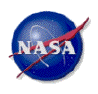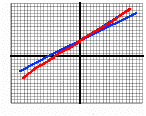+ Text Only Site
+ Non-Flash Version
+ Contact Glenn## Showing the Lift Equation in its Y = mX + b FormNAME__________________________________ CLASS__________

1a. Identify each letter in the lift equation and list the units for each.

Cl = Lift Coefficient (no units)

L = Lift (newtons)

r = Density of Air (kg/m3)

V = Velocity (m/s)

A = Area of Wing (m2)

1b. Identify each letter in the lift equation and list the units for each.

Cl = Lift Coefficient (no units)

a = Angle of Attack (radiians no units)

Clo = Lift Coefficient at a = zero (no units)

2. Write out the two equations for the value of Cl.

Cl = L / (r * V2 / 2 * A).

Cl = 2 * p * a + Clo

3. Rearrange the two equations and solve for L as a function of a.

L = (2 * p * a + Clo) * (r * V2 / 2 * A)

OR

L = (2 * p * r * V2 / 2 * A) * a + (Clo * r * V2 / 2 * A)

Notice this is in the form Y = mX + b.

L = (2 * p * r * V2 / 2 * A) * a + (Clo * r * V2 / 2 * A)

Y = dependent variable, X = independent variable, m = slope, b = Y intercept.

4. Record the values shown:

L = 3,030 newtons

A = 2 square meters

V = 160 km/hr = 44.4 m/sec

r = 1.23 kg/m cubed

a = zero

Clo = 2 * L / r * v squared * A = 1.24

5 & 6

Calculated numbers may vary slightly from those shown below due to round-off errors.

 angle in degrees angles in radians value of lift from Foilsim calculated values of lift -20 -.3491 -2291 -3024 -15 -.2618 -959 -1523 -10 -.1745 372 -1.8 -5 -.08726 1704 1520 0 0 3036 3030 5 .08726 4367 4517 10 .1745 5699 5970 15 .2618 7031 7278 20 .3491 8363 8729The plots are different.

This is due to the fact that the thin airfoil equation contains an approximation that is only good at small values of a . Foilsim does not use this approximation.

7. The value of lift scales linearly as a function of the area of the wing as can be seen by the linear change in the value of lift.

Related Pages:
Standards
Activity
Worksheet
Lesson Index
Aerodynamics Index+ Inspector General Hotline + Equal Employment Opportunity Data Posted Pursuant to the No Fear Act + Budgets, Strategic Plans and Accountability Reports + Freedom of Information Act + The President's Management Agenda + NASA Privacy Statement, Disclaimer, and Accessibility CertificationEditor: Tom Benson NASA Official: Tom Benson Last Updated: Thu, May 13 02:38:38 PM EDT 2021 + Contact Glenn